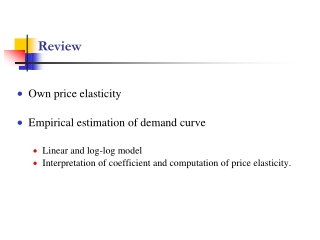# Review - PowerPoint PPT PresentationDownload PresentationReview

ReviewDownload Presentation## Review

- - - - - - - - - - - - - - - - - - - - - - - - - - - E N D - - - - - - - - - - - - - - - - - - - - - - - - - - -
##### Presentation Transcript

1. Review • Own price elasticity • Empirical estimation of demand curve • Linear and log-log model • Interpretation of coefficient and computation of price elasticity.

2. Lecture 11Price Discrimination 1

3. Introduction • Price dispersion – different prices for the same product - is prevalent in the marketplace • The “law of one price” is dead • Example: Microsoft software version and upgrade prices • Example: Prices of 1/3 liter Coca-Cola cans in Germany

4. Prices of 1/3 liter Coca-Cola cans

5. Example: Student rates • Why do software manufacturers have lower rates for students? • One explanation is that they have lower willingness to pay & higher elasticity than business users. • Charging two prices is more profitable

6. Example: Airline pricing • Why do airlines have “first class” rates often 2-3 times higher than cabin? • There is a small group of “elite” customers who are less price elastic than “regular” customers • It is more profitable to charge two prices • The “elites” must be “fenced in” from buying cabin seats by making cabin seats uncomfortable

7. Illustration of benefits of price customization Price (\$) 100 Demand curve Gross Margins (p-c)*q p c=10 0 1000 q

8. Remember the meaning of the demand curve The demand curve is an aggregation of the demands of individual or segment, who have different reservation prices (max. willingness to pay) Price (\$) q 0 Ideally, charge each customer their reservation price (as long as it is above c) – (i) no profitable customer is excluded from buying and (ii) no money left on table.

9. A single price is inefficient Money left on the table Price (\$) 100 Profit (p*-c)q* No trade – deadweight loss p* c=10 0 q 1000

10. Calculation • The demand function is p = 100 – 0.1q • The profit-maximizing price is • The demand at the profit maximizing price is • The profit (gross margins) is

11. Demonstration of benefits using 2 price points Price (\$) Rule of 1/3 for linear demand functions 100 p1* p2* c 0 1000 q1* q2*

12. Calculation p = a – bq q = A – Bp • Assume that we can put a “fence” that prevents higher price customers from paying the lower price, also, c=\$10 • The demand function is q= D(p) = 1000 – 10p • Profit at prices p1 and p2 are • The profit-maximizing prices are • The maximized profit is (p1-c)D(p1)+(p2-c)(D(p2)-D(p1)) p2* =\$40, p1*=\$70 (70-10)*300+(40-10)(600-300)=\$27000

13. Calculation p = a – bq q = A – Bp • In general, in the case of the linear demand, constant variable cost and two prices, the profit-maximizing prices are • p2* = (2c + a)/3, or p2* = (2cB + A)/3B • p1* = (2a + c)/3, or p1* = (2A + cB)/3B

14. This is price discrimination • Charging different prices to different people for the same (or similar) product. • Since “discrimination” has a negative connotation, call it “price customization” • A useful result - Most of the benefit of price customization may be realized with relatively few price points

15. Implementation • Key problem: How do we prevent higher willingness-to-pay customers from paying the price meant for lower willingness-to-pay customers? • Place “fences” that corral customers

16. Next Lecture • Price Discrimination 2 - Implementation